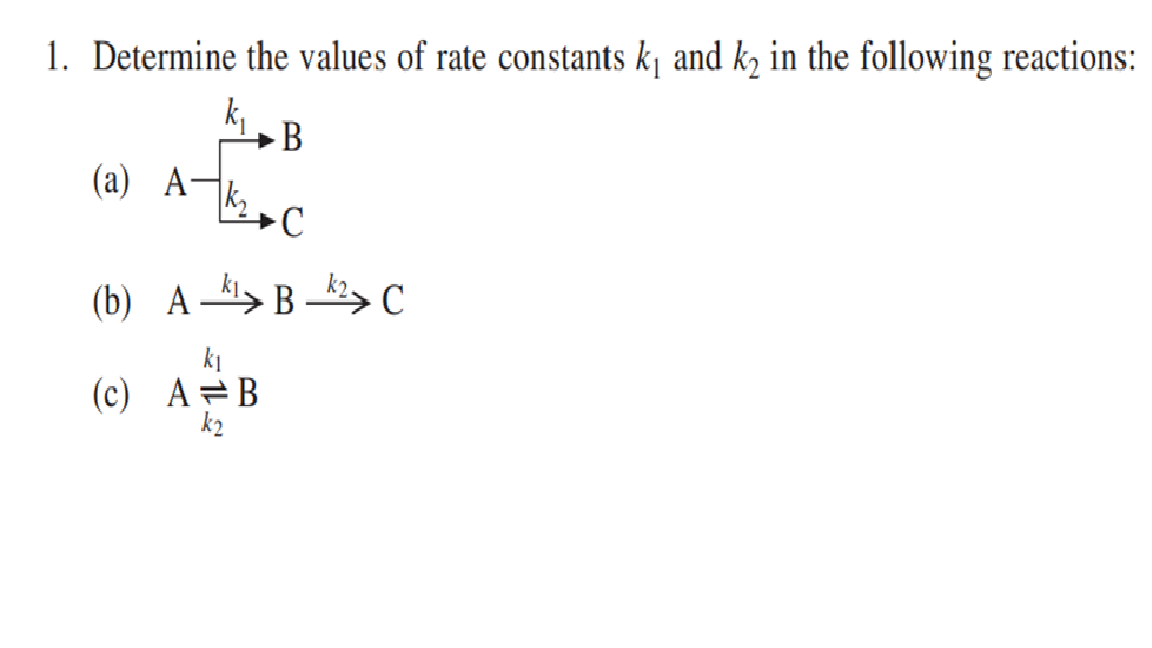# Question Determine the values of rate constants k1 and k2 in thefollowing reactions: (a) A k1 B K2 C (b) A k1 B k2 C (c) A k1 k2B 1. Determine the values of rate constants kļ and k in the following reactions: ki,B (a) Atthac С (b) AB 82C ki (c) A=B k2VSCWMU The Asker · Chemical Engineering

Determine the values of rate constants k1 and k2 in the following reactions: (a) A k1 B K2 C (b) A k1 B k2 C (c) A k1 k2 BTranscribed Image Text: 1. Determine the values of rate constants kļ and k in the following reactions: ki,B (a) Atthac С (b) AB 82C ki (c) A=B k2
More
Transcribed Image Text: 1. Determine the values of rate constants kļ and k in the following reactions: ki,B (a) Atthac С (b) AB 82C ki (c) A=B k2